## Welcome to the Second Life Forums Archive

These forums are CLOSED. Please visit the new forums HERE

# LSL based FORTH interpretor

Alondria LeFay
Registered User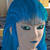Join date: 2 May 2003
Posts: 725
01-31-2005 23:20
Okay, I was bored one day and decided to try something "different" and write a programming language in LSL. While not the first one (SL Lisp was the first), it is (to my knowledge) the first programming language written in LSL that can do more than basic math (although it can do that also). It is modelled after the Forth programming language, although I had to take some liberties in changing some of it's commands (for example, memory mapping would be too slow in LSL, so I cheated an created a string variable type).
It does feature logic (if/else/then, <, >, =, <>, etc) as well as a basic loop (do [blahblah] loop). It allows defining new words - ': test .( Hello World! ) ;'. The language is extendable in that one could also add new core words in LSL and add it to it's dictionary. It also features an exec function, which allows it to execute a hunk of code stored in a string.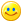First I'll post the list of primitive words it understands:

CODE
`CORE WORDS INPLEMENTED:.            ( N -- , print number on top of stack ).(            ( -- , Prints what is between '.(' and terminated by ')' ).s          ( -- , Prints the stack depth and the stack )+!            ( -- , Prepends a string to a var )!            ( -- , Stores a string into a variable EX: \$( foo ) \$foo ! )!+            ( -- , Appends a string to a var )\$(            ( -- , Notates a string, terminated by ')' )@            ( -- , Prints the value of a string Ex: \$foo @ ):            ( -- , Used to define following word as what follows ended by ; );            ( -- , Used to terminate a word definition )+            ( n1 n2 -- result , Adds n1 to n2 and pushes the result )*            ( n1 n2 -- result , Multiplies n1 to n2 and pushes the result )/            ( n1 n2 -- quotient , Divides n1 by n2 leaving the quotient )?dup        ( n -- n n | 0 , duplicate only if non-zero, '|' means OR ) -            ( n1 n2 -- result, Subtracks n2 from n1 and pushes the result )>            ( n1 n2 -- -1|0 , If n1 is larger than n2, push true (-1) else false (0) )<            ( n1 n2 -- -1|0 , If n1 is smaller than n2 push true else false )=            ( n1 n2 -- -1|0 , If n1 is equal to n2 push true else false )0=            ( n -- -1|0 , If n equals 0, push true else false )0<            ( n -- -1|0 , if n is less than 0 push true else false )1+            ( n -- n+1 , Adds 1 to the top of stack and pushes result )1-            ( n -- n-1 , Subtracts 1 from the top of stack and pushes result )2drop        ( a b -- , remove pair ) 2dup        ( a b -- a b a b , duplicate pair ) 2over        ( a b c d -- a b c d a b , leapfrog pair ) 2swap        ( a b c d -- c d a b , swap pairs ) abs            ( n -- abs(n) , absolute value of n ) and            ( x1 x2 -- x3 , logical bit operator )debug        ( n -- , n switch turns debuger on or off )depth        ( -- n , Pushes the size of the stack )dic            ( n -- , Changes to dictionary n )do            ( n -- , Does what is between 'do' and 'loop' n times )drop        ( a -- , remove item from the stack )dup            ( n -- n n , DUPlicate top of stack )dumpdict    ( -- , Dumps the definitions in the current dictionary)else        ( -- , Does what is between 'else' and 'then' if previous 'if' was false )emit        ( n -- , Displays ASCII character n )empty        ( ... -- , Empties the stack )exec        ( -- , Dumps a string to the run stack )fd            ( n -- , Moves object n units forward )getpos        ( -- x y z , Pushes the position of the object, in movement units )gettime     ( -- n , Pushes the runing time into the stack )gox            ( n -- , move n movement units on the x axis )goy            ( n -- , move n movement units on the y axis )goz            ( n -- , move n movement units on the z axis )grab        ( INTERNAL USE ONLY )if             ( n -- , Does what is between 'if' and 'else' or 'then' if n is true )loop        ( -- , Used to terminate a do )max            ( n1 n2 -- n1|n2 , pushes the larger between n1 and n2 )min            ( n1 n2 -- n1|n2 , pushes the smaller between n1 and n2 )ms            ( n -- , Sleeps for n ms )mod            ( n1 n2 -- remainder , Divides n1 by n2 and leaves the remainder )movescale    ( n -- , Sets the movement units scale )negate        ( n -- -n , negate value, faster then -1 * ) nip            ( a b -- b , remove second item from stack ) onattact    ( -- , Attaches a word to the attach event )onrez        ( -- , Attaches a word to the rez event )ontouch     ( -- , Attachss a word to the touch event )over        ( a b -- a b a , copy second item on stack )pick        ( xu ... x0 u -- xu ... x0 xu )  Copy xu to top of stackpop            ( INTERNAL USE ONLY )push        ( INTERNAL USE ONLY )random        ( n -- [0 - n] , random number from 0 to n )reset        ( -- , Resets the engine )roll        ( xu ... x0 u -- xu-1 ... x0 xu )  Rotate xu to top of stackrot            ( a b c -- b c a , ROTate third item to top ) rotx        ( n -- , Rotates n degrees along the x axis )roty        ( n -- , Rotates n degrees along the y axis )rotz        ( n -- , Rotates n degrees along the z axis )rt            ( n -- , Rotates object n degrees on he z axis )setcolor    ( r g b -- , Sets color of object where number is 0 (none) to 100 (full))swap        ( a b -- b a , swap top two items on stack )then        ( -- , Used to terminate an if ... else ... then clause ) `

Below is a few example words/programs:

CODE
`: hello .( Hello world! ) ;                                    ( -- ) Hello world example: shape dup 360 swap / swap do 2dup rt fd loop 2drop ;      ( n1 n2 -- ) Draw a shape with n2 sides and n1 units length per side.: tosscoin 1 random if .( Heads ) else .( Tales ) then ;     ( -- ) Flips a coin: lt negative rt ;                                            ( n -- ) Turn n degrees to the left: bk negative fd ;                                            ( n -- ) Move n units forward: true -1 ;                                                    ( -- n ) Push true: false 0 ;                                                 ( -- n ) Push false`

One uses the engine by saying the code to process, prepended by 4th. (For example:
CODE
`4th 1 1 + .`

will cause the engine to add 1 and 1 and then print the result.
Agatha Palmerstone
Space Girl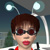Join date: 23 Jan 2005
Posts: 185
02-01-2005 08:07
neat-o!

This will be fun to play with.
Walker Spaight
Raving Correspondent
Join date: 2 Jan 2005
Posts: 281
02-01-2005 08:39
You were bored "one day"? That must have been quite a day.

Really impressive, Alondria, really nice. Where does one get an in-world OS/compiler/whatever one needs to run this language? (And it needs some kind of snappy name, doesn't it? Like LeFORTH or something.)

And are you going to make the LSL source code available?
Chandra Page
Build! Code. Sleep?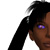Join date: 7 Oct 2004
Posts: 360
02-01-2005 12:45
This is highly disturbing, and I'm completely stunned by the sheer magnitude of geekiness required to accomplish such a task. I commend you on your superior nerdmanship.I must retreat to my secret lair and contemplate how best to use this for evil.
_____________________
Come visit the In Effect main store and café
Drawbridge (160, 81)
Particle effects, fashion, accessories, and coffee!
On the Web at SL Exchange and SL Boutique
Chuck Beckett
Registered User
Join date: 9 Aug 2004
Posts: 84
A Forth interpreter sounds great - where's the script?
02-01-2005 17:18
I suspect a Forth interpreter would be both fun and useful. I don't see the script for the interpreter or info on how to get it though. If you have decided to sell it please let me know how to buy a copy. I want to play with it right this minute!Alondria LeFay
Registered UserJoin date: 2 May 2003
Posts: 725
02-01-2005 19:27
The code, due to it's size and dynamic memory requirements, is split up into several subscripts. The follow is the main script (Named: FORTH.main)

CODE
`// FORTH.main// By Alondria LeFay // Version 0.6// SL Forth - An Second Life Forth Engine// Copyright (C) 2004 Alondria LeFay (alondria@alondria.us)//// This program is free software; you can redistribute it and/or// modify it under the terms of the GNU General Public License// as published by the Free Software Foundation; either version 2// of the License, or (at your option) any later version.//// This program is distributed in the hope that it will be useful,// but WITHOUT ANY WARRANTY; without even the implied warranty of// MERCHANTABILITY or FITNESS FOR A PARTICULAR PURPOSE.  See the// GNU General Public License for more details.//// You should have received a copy of the GNU General Public License// along with this program; if not, write to the Free Software// Foundation, Inc., 59 Temple Place - Suite 330, Boston, MA  02111-1307, USA.integer lhook;integer ich = 0;        // Channel to listen tointeger address = 1;integer cur = 2;string saddress;list cmdstack = [];string define;integer tagdefine = 0;string stringvalue = "";string stringname = "";list dict1 = [    "pop",2,    "push",2,    "drop",2,    "grab",2,    "dup",2,    ".",2,    "depth",2,    "swap",2,    "pick",2,    "roll",2,    "smemfree",2,    ".s",2,    "+",2,    "*",2,    "/",2,    "-",2,    ">",2,    "<",2,    "=",2,    "<>",2,    "0=",2,    "0<",2,    "1+",2,    "1-",2,    "gettime",5,    "debug",5    ];list dict2 = [    "empty",2,    "min",2,    "max",2,    "mod",2,    "negate",2,    "over",2,    "rot",5,    "*/",5,    "2drop",5,    "2dup",5,    "2over",5,    "2swap",5,    "?dup",5,    "abs",5,    "and",5,    "dic",5,    "nip",5,    "random",5,    "emit",3,    "setcolor",4,    "gox",4,    "goy",4,    "goz",4,    "movescale",4,    "getpos",4,    "fd",4,    "rt",4,    "getrot",4,    "rotx",4,    "roty",4,    "rotz",4,    "dumpdict",200,    "reset",50,    "ms",5    ];list dict3 = [    "!",51,    "@",51,    "exec",51,    "!+",51,    "+!",51,    "ontouch",51    ];list dictionary;  integer dic = 200;string text;alInit(integer argi){     if (lhook)     {          llListenRemove(lhook);     }     saddress = (string)address;     lhook = llListen(ich,"","","");     dictionary = dict1 + dict2 + dict3;     dict1 = [];     dict2 = [];     dict3 = [];     llSay(0,"SL-FORTH v0.6 by Alondria LeFay\n" + (string)llGetFreeMemory() + " bytes free in main core\nOk");}integer alIsInt(string foo){     integer tmpi = (integer)foo;    if (foo == (string)tmpi)    {        return TRUE;    }    return FALSE;}string alPopCmd(){    if (llGetListLength(cmdstack) == 0)    {        return "";    }    string foo = llList2String(cmdstack,0);    cmdstack = llDeleteSubList(cmdstack,0,0);    return foo;}alParseText(){    string tmps = alPopCmd();    if (tmps == "" || tmps == ")")    {        if (tagdefine == 2)        {            llSay(0,text);        }        else if (tagdefine == 3)        {            stringvalue = text;        }        tagdefine = 0;        text = "";        if (tmps == ")")        {            alForth();        }        return;    }    text = text + " " + tmps;    alParseText();    return;}alForth(){    string foo = alPopCmd();    if (foo == "")    {        llSay(0,"Ok-stop");        return;    }    if (tagdefine == 1)    {        if (foo == ";")        {            llMessageLinked(-1,dic,"define " + define,saddress);            tagdefine = 0;            define = "";            return;        }        define = define + " " + foo;        alForth();        return;    }    if (foo == "mmemfree")    {        llSay(0,"FORTH.main = " + (string)llGetFreeMemory() + " bytes");        return;    }    else if (foo == "do")    {        llMessageLinked(-1,cur,"passstack","");        llSleep(.5);        string tmps = alPopCmd();        string foo;        while (tmps != "loop")        {            foo = foo + " " + tmps;            tmps = alPopCmd();        }        llMessageLinked(-1,300,"do " + foo,saddress);        return;    }    else if (foo == "if")    {        llMessageLinked(-1,cur,"passstack","");        string tmps = alPopCmd();        string foo;        while (tmps != "then")        {            foo = foo + " " + tmps;            tmps = alPopCmd();        }        llMessageLinked(-1,300,"if " + foo,saddress);        return;    }    if (alIsInt(foo))    {        if (cur < 50 )         {            llMessageLinked(-1,cur,"push " + foo,saddress);        }        else         {            llMessageLinked(-1,1,"push " + foo,saddress);        }        return;    }    integer tmpi = llListFindList(dictionary, [ foo ]);    if (tmpi != -1)    {        tmpi = tmpi + 1;        tmpi = llList2Integer(dictionary,tmpi);        if (tmpi != cur && tmpi < 50)        {            llMessageLinked(-1,cur,"passstack",saddress);            llSleep(.5);        }        cur = tmpi;        llMessageLinked(-1,tmpi,foo + " " + stringname + " " + stringvalue,saddress);        stringname = "";        stringvalue = "";        return;    }    else if (foo == ":")    {        tagdefine = 1;        alForth();        return;    }    else if (foo == ".(")    {        tagdefine = 2;        alParseText();    }    else if (foo == "\$(")    {        tagdefine = 3;        alParseText();        return;    }    else if (llGetSubString(foo,0,0) == "\$")    {        stringname = foo;        alForth();        return;    }    else    {        llMessageLinked(-1,dic,"call " + foo + " " + stringname + " " + stringvalue,saddress);        stringname = "";        stringvalue = "";    }    return;}alParse(integer channel, string name, key id, string message){    if (channel != 0 && channel != 1)    {        return;    }    list args;    args=llParseString2List(message, [" "],[":"]);    string obj=llList2String(args,0);    args=llDeleteSubList(args,0,0);     if (obj == "4th")    {        cmdstack = cmdstack + args;        alForth();        return;    }    else if (obj == "4thp")    {        cmdstack = args + cmdstack;        alForth();        return;    }    else if (obj == "dict")    {        dic = llList2Integer(args,0);        return;    }    else if (obj == "unknown")    {        llSay(0,"Error: " + llList2String(args,0) + " is undefined.");        cmdstack = [];        return;    }    return;}default{    on_rez(integer argi)    {    }    state_entry()    {        alInit(0);    }    listen(integer channel, string name, key id, string message)    {        alParse(channel, name, id, message);    }    link_message(integer sender_num, integer num, string str, key id)    {        if (num == 1)        {            if (str == "ok")            {                alForth();                return;            }        }        alParse(num,"link",id,str);    } }`
Alondria LeFay
Registered UserJoin date: 2 May 2003
Posts: 725
02-01-2005 19:38
The following sub-script (FORTH.stack) contains the main stack manipulation functions

CODE
`// FORTH.stack// Purpose: Main stack manipulation functions// Copyright 2004 By Alondria LeFay// Version 0.6integer lhook;integer address = 2;string saddress;list stack;string ret;alInit(integer argi){    saddress = (string)address;     if (lhook)     {          llListenRemove(lhook);     }}// + ( n1 n2 -- n3 )// Adds n1 to n2 to make n3alPlus(){    alPush(alPop() + alPop());}// * ( n1 n2 -- n3 )// Multiplies n1 to n2 and pushes n3alStar(){    alPush(alPop() * alPop());}// .S ( -- )// Displays the stackalDotS(){    string tmps;    integer slength = llGetListLength(stack);    tmps = "[" + (string)slength + "] ";    integer i = slength;    for (i = slength; i > -1; i = i - 1)    {        tmps = tmps + llList2String(stack,i) + " ";    }    llSay(0,tmps);}// Roll ( xu ... x0 u -- xu-1 ... x0 xu )// Rotates xu to TOSalRoll(){    alPush(alYank(alPop()));}// Yank ( xu ... x0 u -- drop xu)// Pop's the u'th element and returns it (not pushed)integer alYank(integer int){    integer tmpi = llList2Integer(stack,int);    stack = llDeleteSubList(stack,int,int);    return tmpi;}// Pick ( xu ... x0 u -- xu ... x0 xu)// Copy xu to TOSalPick(){    integer tmpi = alPop();    tmpi = llList2Integer(stack,tmpi);    alPush(tmpi);}// Pop ( x -- )// Pops the TOS off and returns itinteger alPop(){    integer int = llList2Integer(stack,0);    //stack = llDeleteSubList(stack,0,0);    alDrop();    return int;}// Swap ( x1 x2 -- x2 x1 )// Switchs possitions of x1 and x2alSwap(){    integer tmpi = alPop();    integer tmpi2 = alPop();    stack = llListInsertList(stack, [ tmpi2, tmpi ], 0);    return;}// Depth ( -- x )// Pushes length of stack to TOSalDepth(){    alPush(llGetListLength(stack));}// Dup ( x -- x x )// Copies TOSalDup(){    alPush(alGrab());}// Drop ( x -- )// Drop TOS from stackalDrop(){    stack = llDeleteSubList(stack,0,0);}// Grab ( x -- x )// Returns TOS but does not popinteger alGrab(){    return llList2Integer(stack,0);}// . ( x -- )// Prints TOS and drops italDot(){    integer int = llList2Integer(stack,0);    stack = llDeleteSubList(stack,0,0);    llSay(0,(string)int);}// Push ( -- x )// Pushes an integer to TOSalPush(integer int){    stack = llListInsertList(stack, [ int ], 0);}alParse(integer channel, string name, key id, string message){    if (channel == address || channel == 0 || channel == 100)    {            list args;        args=llParseString2List(message, [" "],[":"]);        string cmd=llList2String(args,0);        args=llDeleteSubList(args,0,0);        if (cmd == "updatestack")        {            stack = args;            return;        }        if (cmd == "passstack")        {            llMessageLinked(-1,100,"updatestack " + llDumpList2String(stack," "),saddress);            return;        }        if (cmd == ".")        {            alDot();            return;        }        else if (cmd == "pop")        {            string tmps = id;            integer tmpi = (integer)tmps;            llMessageLinked(-1,tmpi,(string)alPop(),saddress);            return;        }        else if (cmd == "push")        {            alPush(llList2Integer(args,0));            return;        }        else if (cmd == "drop")        {            alDrop();            return;        }        else if (cmd == "grab")        {            string tmps = id;            integer tmpi = (integer)tmps;            tmps = (string)alGrab();            llMessageLinked(-1,tmpi, tmps ,(string)saddress);            return;        }        else if (cmd == "dup")        {            alDup();            return;        }        else if (cmd == "depth")        {            alDepth();            return;        }        else if (cmd == "swap")        {            alSwap();            return;        }        else if (cmd == "pick")        {            alPick();            return;        }        else if (cmd == "roll")        {            alRoll();            return;        }        else if (cmd == "smemfree")        {            llSay(0,"Stack memfree: " + (string)llGetFreeMemory());            return;        }        if (cmd == ".s")        {            alDotS();            return;        }        else if (cmd == "+")        {            alPlus();            return;        }        else if (cmd == "*")        {            alStar();            return;        }        else if (cmd == "-")        {            integer n2 = alPop();            integer n1 = alPop();            alPush(n1 - n2);            return;        }        else if (cmd == "/")        {            integer n2 = alPop();            integer n1 = alPop();            alPush(n1 / n2);            return;        }        else if (cmd == ">")        {            integer n2 = alPop();            integer n1 = alPop();            if (n1 > n2)            {                alPush(-1);            }            else            {                alPush(0);            }            return;        }        else if (cmd == "<")        {            integer n2 = alPop();            integer n1 = alPop();            if (n1 < n2)            {                alPush(-1);            }            else            {                alPush(0);            }            return;        }        else if (cmd == "=")        {            integer n2 = alPop();            integer n1 = alPop();            if (n1 == n2)            {                alPush(-1);            }            else            {                alPush(0);            }            return;        }               else if (cmd == "0=")        {            if (alPop() == 0)            {                alPush(-1);            }            else            {                alPush(0);            }            return;        }        else if (cmd == "0<")        {            if (alPop() < 0)            {                alPush(-1);            }            else            {                alPush(0);            }            return;        }        if (cmd == "1+")         {            alPush(alPop() + 1);            return;        }        else if (cmd == "<>")        {            integer n2 = alPop();            integer n1 = alPop();            if (n1 != n2)            {                alPush(-1);            }            else            {                alPush(0);            }            return;        }        else if (cmd == "1-")        {            alPush(alPop() - 1);            return;        }        else if (cmd == "empty")        {            stack = [];            return;        }        else if (cmd == "max")        {            integer n1 = alPop();            integer n2 = alPop();            if (n1 > n2)            {                alPush(n1);                return;            }            alPush(n2);            return;        }        else if (cmd == "min")        {            integer n1 = alPop();            integer n2 = alPop();            if (n1 < n2)            {                alPush(n1);                return;            }            alPush(n2);            return;        }        else if (cmd == "mod")        {            integer n2 = alPop();            integer n1 = alPop();            alPush(n1 % n2);            return;        }        else if (cmd == "negate")        {            alPush(alPop() * -1);            return;        }        if (cmd == "over")        {            integer n2 = alPop();            integer n1 = alPop();            stack = [ n1 , n2 , n1 ] + stack;            return;        }        return;    }    return;}default{    on_rez(integer argi)    {        alInit(argi);    }    state_entry()    {        alInit(0);    }    listen(integer channel, string name, key id, string message)    {        alParse(channel, name, id, message);    }    link_message(integer sender_num, integer num, string str, key id)    {        alParse(num,"link",id,str);        string tmps = (string)id;        if (num == address && str != "passstack")        {            llMessageLinked(-1,(integer)tmps,"ok",saddress);        }    } }`

/edited due to botch copy and paste or something..... original would not compile.
Alondria LeFay
Registered UserJoin date: 2 May 2003
Posts: 725
FORTH.stack2
02-01-2005 19:56
The following is more stack based core words:

CODE
`// FORTH.stack.2// Copyright 2004 By Alondria LeFay// Version 0.6integer lhook;integer address = 5;string saddress;list stack;string ret;alInit(integer argi){    saddress = (string)address;     if (lhook)     {          llListenRemove(lhook);     }}// Pop ( x -- )// Pops the TOS off and returns itinteger alPop(){    integer int = llList2Integer(stack,0);    //stack = llDeleteSubList(stack,0,0);    alDrop();    return int;}// Swap ( x1 x2 -- x2 x1 )// Switchs possitions of x1 and x2alSwap(){    integer tmpi = alPop();    integer tmpi2 = alPop();    stack = llListInsertList(stack, [ tmpi2, tmpi ], 0);    return;}// Dup ( x -- x x )// Copies TOSalDup(){    alPush(alGrab());}// Drop ( x -- )// Drop TOS from stackalDrop(){    stack = llDeleteSubList(stack,0,0);}// Grab ( x -- x )// Returns TOS but does not popinteger alGrab(){    return llList2Integer(stack,0);}// Push ( -- x )// Pushes an integer to TOSalPush(integer int){    stack = llListInsertList(stack, [ int ], 0);}alParse(integer channel, string name, key id, string message){    if (channel == address || channel == 0 || channel == 100)    {            list args;        args=llParseString2List(message, [" "],[":"]);        string cmd=llList2String(args,0);//string obj=llList2String(args,0);        args=llDeleteSubList(args,0,0);//llWhisper(0,"In FORTH.stack -> " + cmd);        if (cmd == "updatestack")        {            stack = args;            return;        }        if (cmd == "passstack")        {            llMessageLinked(-1,100,"updatestack " + llDumpList2String(stack," "),saddress);            return;        }        else if (cmd == "push")        {            alPush(llList2Integer(args,0));            return;        }        if (cmd == "rot")        {            integer n3 = alPop();            integer n2 = alPop();            integer n1 = alPop();            stack = [ n1 , n3, n2 ] + stack;            return;        }        else if (cmd == "*/")        {            integer n3 = alPop();            integer n2 = alPop();            integer n1 = alPop();            alPush((n1 * n2) / n3);            return;        }        else if (cmd == "2drop")        {            alDrop();            alDrop();            return;        }        else if (cmd == "2dup")        {             integer n2 = alPop();            integer n1 = alPop();            stack = [ n2, n1, n2, n1 ] + stack;            return;        }        else if (cmd == "2over")        {            integer n4 = alPop();            integer n3 = alPop();            integer n2 = alPop();            integer n1 = alPop();            stack = [ n2, n1, n4, n3, n2, n1 ] + stack;            return;        }        else if (cmd == "2swap")        {            integer n4 = alPop();            integer n3 = alPop();            integer n2 = alPop();            integer n1 = alPop();            stack = [ n2, n1, n4, n3 ] + stack;            return;        }        else if (cmd == "dic")        {            llMessageLinked(-1,1,"dict " + (string)alPop(),saddress);            return;        }        if (cmd == "?dup")        {            integer n1 = alGrab();            if (n1 != 0)            {                alPush(n1);            }            return;        }        else if (cmd == "abs")        {            integer n1 = alPop();            alPush(llAbs(n1));            return;        }        else if (cmd == "nip")        {            integer n2 = alPop();            integer n1 = alPop();            alPush(n2);            return;        }        else if (cmd == "and")        {            integer n2 = alPop();            integer n1 = alPop();            alPush(n1 & n2);            return;        }        else if (cmd == "random")        {            alPush(llFloor(llFrand(alPop() + 1)));            return;        }        else if (cmd == "gettime")        {            alPush((integer)(llGetTime() * 100));            return;        }        else if (cmd == "ms")        {            llSleep((alPop() / 100));            return;        }        else if (cmd == "debug")        {            integer tmpi = alPop();            if (tmpi == 0)            {                llSetScriptState("OBJ.debug", FALSE);            }            else            {                llSetScriptState("OBJ.debug",TRUE);            }            return;        }        return;    }    return;}default{    on_rez(integer argi)    {        alInit(argi);    }    state_entry()    {        alInit(0);    }    listen(integer channel, string name, key id, string message)    {        alParse(channel, name, id, message);    }    link_message(integer sender_num, integer num, string str, key id)    {        alParse(num,"link",id,str);        string tmps = (string)id;        if (num == address && str != "passstack")        {            llMessageLinked(-1,(integer)tmps,"ok",saddress);        }    } }`
Alondria LeFay
Registered UserJoin date: 2 May 2003
Posts: 725
FORTH.dictionary
02-01-2005 19:58
The following stores/calls user created words:

CODE
`// FORTH.dictionary// Copyright 2004 By Alondria LeFay// Version 0.6integer lhook;integer address = 200;string saddress;list index = [];list value = [];alInit(integer argi){     if (lhook)     {          llListenRemove(lhook);     }     saddress = (string)address;}alParse(integer channel, string name, key id, string message){    if (channel == 0 || channel == address)    {        string tooo = (string)id;        if ((integer)tooo != 1)        {             return;        }        list args;        args=llParseString2List(message, [" "],[":"]);        string cmd=llList2String(args,0);        args=llDeleteSubList(args,0,0);        if (cmd == "define")        {            string tmps = llList2String(args,0);            args = llDeleteSubList(args,0,0);             index = index + [ tmps ];            tmps = llDumpList2String(args, " ");            value = value + [ tmps ];            tmps = (string)id;            llMessageLinked(-1,(integer)tmps,"ok",saddress);            return;        }        if (cmd == "call")        {            string tmps = llList2String(args,0);            integer tmpi = llListFindList(index, [ tmps ]);            if (tmpi == -1)            {                tmps = (string)id;                llMessageLinked(-1,(integer)tmps,"unknown " + llList2String(args,0),saddress);                return;            }            else            {                tmps = (string)id;                llMessageLinked(-1,(integer)tmps,"4thp " + llList2String(value,tmpi),saddress);                return;            }            return;        }        else if (cmd == "see")        {            string tmps = llList2String(args,0);            integer tmpi = llListFindList(index, [ tmps ]);            if (tmpi == -1)            {                tmps = (string)id;                llMessageLinked(-1,(integer)tmps,"unknown " + llList2String(args,0),saddress);                return;            }            else            {                llSay(0,tmps + " : " + llList2String(value,tmpi));                return;            }        }        else if (cmd == "dumpdic");        {            index = [];            value = [];            string tmps = (string)id;            llMessageLinked(-1,(integer)tmps,"ok",saddress);            return;        }        return;    }    return;}default{    on_rez(integer argi)    {        alInit(argi);    }    state_entry()    {        alInit(0);    }    listen(integer channel, string name, key id, string message)    {        alParse(channel, name, id, message);    }    link_message(integer sender_num, integer num, string str, key id)    {        alParse(num,"link",id,str);    } }`
Alondria LeFay
Registered UserJoin date: 2 May 2003
Posts: 725
FORTH.string
02-01-2005 20:36
The following is the sub-script that handles string variables:

CODE
`// FORTH.string// Copyright 2004 By Alondria LeFay// Version 0.6integer lhook;integer address = 51;string saddress;list index = [];list value = [];alInit(integer argi){     if (lhook)     {          llListenRemove(lhook);     }     saddress = (string)address;}alParse(integer channel, string name, key id, string message){    if (channel == address || channel == 0 )    {            list args;        args=llParseString2List(message, [" "],[":"]);        string cmd=llList2String(args,0);        args=llDeleteSubList(args,0,0);        string var = llList2String(args,0);        if (cmd == "ontouch")        {            if (llGetSubString(var,0,0) == "\$")            {                integer tmpi = llListFindList(index, [ var ]);                if (tmpi != -1)                {                           string val = llList2String(value, tmpi);                    llMessageLinked(-1,401,"ontouch " + val,saddress);                    return;                }                else                {                    return;                }            }            else            {                llMessageLinked(-1,401,"ontouch " + llDumpList2String(args, " "),saddress);                return;            }        }        if (llGetSubString(var,0,0) != "\$")        {             llSay(0,"Error: No variable name given.");             return;        }        if (cmd == "!")        {            args = llDeleteSubList(args,0,0);            string val = llDumpList2String(args," ");            integer tmpi = llListFindList(index, [ var ]);            if (tmpi != -1)            {                index = llDeleteSubList(index,tmpi,tmpi);                value = llDeleteSubList(value,tmpi,tmpi);            }            index = index + [ var ];            value = value + [ val ];            return;        }        else if (cmd == "@")        {            integer tmpi = llListFindList(index, [ var ]);            if (tmpi == -1)            {                return;            }            llSay(0,llList2String(value,tmpi));        }        else if (cmd == "exec")        {            integer tmpi = llListFindList(index, [ var ]);            if (tmpi == -1)            {                return;            }            llMessageLinked(-1,1,"4thp " + llList2String(value,tmpi),saddress);        }        else if (cmd == "!+")        {            integer tmpi = llListFindList(index, [ var ]);            if (tmpi == -1)            {                return;            }            args = llDeleteSubList(args,0,0);            string val = llList2String(value, tmpi) + " " + llDumpList2String(args," ");            index = llDeleteSubList(index,tmpi,tmpi);            value = llDeleteSubList(value,tmpi,tmpi);                         index = index + [ var ];            value = value + [ val ];            return;        }        else if (cmd == "+!")        {            integer tmpi = llListFindList(index, [ var ]);            if (tmpi == -1)            {                return;            }            args = llDeleteSubList(args,0,0);            string val = llDumpList2String(args," ") + " " + llList2String(value, tmpi);            index = llDeleteSubList(index,tmpi,tmpi);            value = llDeleteSubList(value,tmpi,tmpi);                         index = index + [ var ];            value = value + [ val ];            return;              }        return;    }        return;}default{    on_rez(integer argi)    {        alInit(argi);    }    state_entry()    {        alInit(0);    }    listen(integer channel, string name, key id, string message)    {        alParse(channel, name, id, message);    }    link_message(integer sender_num, integer num, string str, key id)    {        alParse(num,"link",id,str);        string tmps = (string)id;        if (num == address && str != "passstack")        {            llMessageLinked(-1,(integer)tmps,"ok",saddress);        }    } }`
Alondria LeFay
Registered UserJoin date: 2 May 2003
Posts: 725
FORTH.looper
02-01-2005 20:38
This subscript deals with loops and if/else/then blocks:

CODE
`// FORTH.looper// Copyright 2004 By Alondria LeFay// Version 0.6integer lhook;integer address = 300;string saddress;list stack;string ret;alInit(integer argi){     if (lhook)     {          llListenRemove(lhook);     }     saddress = (string)address;}// Push ( -- x )// Pushes an integer to TOSalPush(integer int){    stack = llListInsertList(stack, [ int ], 0);}// Drop ( x -- )// Drop TOS from stackalDrop(){    stack = llDeleteSubList(stack,0,0);}// Pop ( x -- )// Pops the TOS off and returns itinteger alPop(){    integer int = llList2Integer(stack,0);    //stack = llDeleteSubList(stack,0,0);    alDrop();    return int;}alParse(integer channel, string name, key id, string message){    if (channel == address || channel == 0 || channel == 100)    {            list args;        args=llParseString2List(message, [" "],[":"]);        string cmd=llList2String(args,0);        args=llDeleteSubList(args,0,0);        if (cmd == "updatestack")        {            stack = args;            return;        }        if (cmd == "passstack")        {            llMessageLinked(-1,100,"updatestack " + llDumpList2String(stack," "),saddress);            return;        }        else if (cmd == "push")        {            alPush(llList2Integer(args,0));            return;        }        else if (cmd == "do")        {            integer tmpi = alPop();            integer i;            string str = llDumpList2String(args," ");            string foo;            for (i = 0; i < tmpi; i = i + 1)            {                foo = foo + " " + str;            }            llMessageLinked(-1,100,"updatestack " + llDumpList2String(stack," "),saddress);            llMessageLinked(-1,1,"4thp " + foo,saddress);            return;        }        else if (cmd == "if")        {            integer i = alPop();            llMessageLinked(-1,100,"updatestack " + llDumpList2String(stack," "),saddress);            message = llDumpList2String(args," ");            args = llParseString2List(message, ["else"],[""]);            if (i != 0)            {                llMessageLinked(-1,1,"4thp " + llList2String(args,0),saddress);            }            else            {                llMessageLinked(-1,1,"4thp " + llList2String(args,1),saddress);            }            return;        }        return;    }    return;}default{    on_rez(integer argi)    {        alInit(argi);    }    state_entry()    {        alInit(0);    }    listen(integer channel, string name, key id, string message)    {        alParse(channel, name, id, message);    }    link_message(integer sender_num, integer num, string str, key id)    {        alParse(num,"link",id,str);    } }`
Alondria LeFay
Registered UserJoin date: 2 May 2003
Posts: 725
FORTH.sl
02-01-2005 20:40
Second Life word set library:

CODE
`// FORTH.sl// SL based core words.// Copyright 2004 by Alondria LeFay// Version 0.6integer lhook;integer address = 4;list stack;string saddress;integer movescale = 10;alInit(integer argi){     if (lhook)     {          llListenRemove(lhook);     }     saddress = (string)address;}// Pop ( x -- )// Pops the TOS off and returns itinteger alPop(){    integer int = llList2Integer(stack,0);    //stack = llDeleteSubList(stack,0,0);    alDrop();    return int;}// Drop ( x -- )// Drop TOS from stackalDrop(){    stack = llDeleteSubList(stack,0,0);}// Push ( -- x )// Pushes an integer to TOSalPush(integer int){    stack = llListInsertList(stack, [ int ], 0);}alParse(integer channel, string name, key id, string message){    if (channel == address || channel == 0 || channel == 100)    {            list args;        args=llParseString2List(message, [" "],[":"]);        string cmd=llList2String(args,0);        args=llDeleteSubList(args,0,0);        if (cmd == "updatestack")        {            stack = args;            return;        }        if (cmd == "passstack")        {            llMessageLinked(-1,100,"updatestack " + llDumpList2String(stack," "),saddress);            return;        }        else if (cmd == "push")        {            alPush(llList2Integer(args,0));            return;        }        else if (cmd == "setcolor")        {            float f3 = (float)alPop() / 100.0;            float f2 = (float)alPop() / 100.0;            float f1 = (float)alPop() / 100.0;            llSetColor(<f1, f2, f3>, ALL_SIDES);            return;        }        else if (cmd == "gox")        {            vector pos = llGetPos();            float x = pos.x + (float)(alPop() / movescale);            pos.x = x;            llSetPos(pos);            return;        }        else if (cmd == "goy")        {            vector pos = llGetPos();            float x = pos.y + (float)(alPop() / movescale);            pos.y = x;            llSetPos(pos);            return;        }        else if (cmd == "goz")        {            vector pos = llGetPos();            float x = pos.z + (float)(alPop() / movescale);            pos.z = x;            llSetPos(pos);            return;            }        else if (cmd == "movescale")        {            movescale = alPop();            return;        }        else if (cmd == "getpos")        {            vector pos = llGetPos();            alPush((integer)(pos.x * movescale));            alPush((integer)(pos.y * movescale));            alPush((integer)(pos.z * movescale));            return;        }        else if (cmd == "fd")        {               vector foo = llRot2Fwd(llGetRot());            foo = foo * (float)(alPop() / (float)movescale);            foo = llGetPos() + foo;             llSetPos(foo);        }        if (cmd == "rt")        {            vector rot = (RAD_TO_DEG * llRot2Euler(llGetRot()));            rot.z = rot.z + alPop();            rot = rot * DEG_TO_RAD;            llSetRot(llEuler2Rot(rot));        }        else if (cmd == "getrot")        {            vector rot = (RAD_TO_DEG * llRot2Euler(llGetRot()));            alPush((integer)(rot.x));            alPush((integer)(rot.y));            alPush((integer)(rot.z));            return;        }        else if (cmd == "rotx")        {            vector rot = (RAD_TO_DEG * llRot2Euler(llGetRot()));            rot.x = alPop();            rot = rot * DEG_TO_RAD;            llSetRot(llEuler2Rot(rot));            return;        }        else if (cmd == "roty")        {            vector rot = (RAD_TO_DEG * llRot2Euler(llGetRot()));            rot.y = alPop();            rot = rot * DEG_TO_RAD;            llSetRot(llEuler2Rot(rot));            return;        }        else if (cmd == "rotz")        {            vector rot = (RAD_TO_DEG * llRot2Euler(llGetRot()));            rot.z = alPop();            rot = rot * DEG_TO_RAD;            llSetRot(llEuler2Rot(rot));            return;        }                return;    }    return;}default{    on_rez(integer argi)    {        alInit(argi);    }    state_entry()    {        alInit(0);    }    listen(integer channel, string name, key id, string message)    {        alParse(channel, name, id, message);    }    link_message(integer sender_num, integer num, string str, key id)    {        alParse(num,"link",id,str);        string tmps = (string)id;        if (num == address && str != "passstack")        {            llMessageLinked(-1,(integer)tmps,"ok",saddress);        }    } }`
Alondria LeFay
Registered UserJoin date: 2 May 2003
Posts: 725
FORTH.events
02-01-2005 20:43
A rough event driver for SL Forth. It still needs most of the events to be implemented, however I am posting what it is thus far:

CODE
`// FORTH.events// Copyright 2004 by Alondria LeFay// Version 0.1ainteger lhook;integer address = 401;string saddress = "";string ontouch = "";string onattach = "";string onrez = "";alInit(integer argi){     if (lhook)     {          llListenRemove(lhook);     }     saddress = (string)address;}alEvent(string foo){    if (foo == "")    {        return;    }    llMessageLinked(-1,1,"4thp " + foo,saddress);    return;}alParse(integer channel, string name, key id, string message){    if (channel == 0 || channel == address)    {        list args;        args=llParseString2List(message, [" "],[":"]);        string cmd=llList2String(args,0);        args=llDeleteSubList(args,0,0);        if (cmd == "ontouch")        {            ontouch = llDumpList2String(args," ");            return;        }        else if (cmd == "onrez")        {            onrez = llDumpList2String(args, " ");            return;        }        else if (cmd == "onattach")        {            onattach = llDumpList2String(args, " ");            return;        }        return;    }    return;}default{    on_rez(integer argi)    {        alEvent(onrez);    }    state_entry()    {        alInit(0);    }    listen(integer channel, string name, key id, string message)    {        alParse(channel, name, id, message);    }    link_message(integer sender_num, integer num, string str, key id)    {        alParse(num,"link",id,str);    }     touch_start(integer num)    {        llMessageLinked(-1,51,"! \$key " + (string)llDetectedKey(0),saddress);        alEvent(ontouch);    }    attach(key tmpk)    {        llMessageLinked(-1,51,"! \$key " + (string)tmpk,saddress);        alEvent(onattach);    }}`
Alondria LeFay
Registered UserJoin date: 2 May 2003
Posts: 725
FORTH.emit
02-01-2005 20:47
Forth's emit command in LSL:

CODE
`// FORTH.emit// Copyright 2004 by Alondria LeFay// Version 0.6integer lhook;integer address = 3;list stack;string saddress;list ascii1 = ["","","","","","","","","","","","","","","","","","","","","","","","","","","","","","",""];list ascii2 = [" ","!","quote","#","\$","%","&","'","(",")","*","+",",","-",".","/",                "0","1","2","3","4","5","6","7","8","9",":",";","<","=",">","?","@"];list ascii3 = ["A","B","C","D","E","F","G","H","I","J","K","L","M","N","O","P","Q","R","S","T","U","V",                "W","X","Y","Z","[","\\","]","^","_","`"];list ascii4 = ["a","b","c","d","e","f","g","h","i","j","k","l","m","n","o","p","q","r","s","t","u","v",                "w","x","y","z","{","|","}","~","DEL"];list ascii;alInit(integer argi){     if (lhook)     {          llListenRemove(lhook);     }     saddress = (string)address;     ascii = ascii1 + ascii2 + ascii3 + ascii4;     ascii1 = [];     ascii2 = [];     ascii3 = [];     ascii4 = [];}// Pop ( x -- )// Pops the TOS off and returns itinteger alPop(){    integer int = llList2Integer(stack,0);    //stack = llDeleteSubList(stack,0,0);    alDrop();    return int;}// Drop ( x -- )// Drop TOS from stackalDrop(){    stack = llDeleteSubList(stack,0,0);}// Push ( -- x )// Pushes an integer to TOSalPush(integer int){    stack = llListInsertList(stack, [ int ], 0);}alParse(integer channel, string name, key id, string message){    if (channel == address || channel == 0 || channel == 100)    {            list args;        args=llParseString2List(message, [" "],[":"]);        string cmd=llList2String(args,0);        args=llDeleteSubList(args,0,0);        if (cmd == "updatestack")        {            stack = args;            return;        }        if (cmd == "passstack")        {            llMessageLinked(-1,100,"updatestack " + llDumpList2String(stack," "),saddress);            return;        }        else if (cmd == "push")        {            alPush(llList2Integer(args,0));            return;        }        else if (cmd == "setcolor")        {            float f1 = (float)alPop() / 100.0;            float f2 = (float)alPop() / 100.0;            float f3 = (float)alPop() / 100.0;            llSetColor(<f1, f2, f3>, ALL_SIDES);            return;        }        else if (cmd == "emit")        {            integer n1 = alPop();            llSay(0,llList2String(ascii,n1));            return;        }        return;    }    return;}default{    on_rez(integer argi)    {        alInit(argi);    }    state_entry()    {        alInit(0);    }    listen(integer channel, string name, key id, string message)    {        alParse(channel, name, id, message);    }    link_message(integer sender_num, integer num, string str, key id)    {        alParse(num,"link",id,str);        string tmps = (string)id;        if (num == address)        {            llMessageLinked(-1,(integer)tmps,"ok",saddress);        }    } }`
Alondria LeFay
Registered UserJoin date: 2 May 2003
Posts: 725
FORTH.nonstack
02-01-2005 20:51
Subscript to handle words that don't require any stack manipulation to speed up the engine by not passing the stack. Currently only has reset and resettime.

CODE
`// FORTH.nonstack// Copyright 2004 by Alondria LeFay// Version 0.6integer lhook;integer address = 50;string saddress;alInit(integer argi){     if (lhook)     {          llListenRemove(lhook);     }     saddress = (string)address;}alParse(integer channel, string name, key id, string message){    if (channel == address || channel == 0 || channel == 100)    {            list args;        args=llParseString2List(message, [" "],[":"]);        string cmd=llList2String(args,0);        //string obj=llList2String(args,0);        args=llDeleteSubList(args,0,0);        if (cmd == "reset")          {              integer max = llGetInventoryNumber(INVENTORY_SCRIPT);              integer i;              string me = llGetScriptName();              for (i = 0; i < max; i = i + 1)              {                  string script = llGetInventoryName(INVENTORY_SCRIPT, i);                if (script != me)                {                      llResetOtherScript(script);                  }                                }              llResetScript();          }          else if (cmd == "resettime")          {              llResetTime();              return;          }        return;    }        return;}default{    on_rez(integer argi)    {        alInit(argi);    }    state_entry()    {        alInit(0);    }    listen(integer channel, string name, key id, string message)    {        alParse(channel, name, id, message);    }    link_message(integer sender_num, integer num, string str, key id)    {        alParse(num,"link",id,str);        string tmps = (string)id;        if (num == address)        {            llMessageLinked(-1,(integer)tmps,"ok",saddress);        }    } }`
Hiro Pendragon
bye bye f0rums!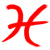Join date: 22 Jan 2004
Posts: 5,905
02-02-2005 01:51
God, wow, I wish I were bored enough to have time to do something like this!

If this works, great job! I'd love to see how this performs. This could allow newbies to write simple instructions on notecards and see them scripted.
_____________________
Hiro Pendragon
------------------
http://www.involve3d.com - Involve - Metaverse / Emerging Media Studio

Visit my SL blog: http://secondtense.blogspot.com
Issarlk Chatnoir
Cross L. apologist.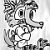Join date: 3 Oct 2004
Posts: 424
02-02-2005 04:15
That's really cool. Doing programs without need of compiling them is interesting.
_____________________
Vincit omnia Chaos
From: Flugelhorn McHenry
Anyway, ignore me, just listen to the cow
Marina McTeague
Registered User
Join date: 20 Jan 2005
Posts: 18
02-02-2005 14:10
You're crazy!

Excellent job.
Hiro Pendragon
bye bye f0rums!Join date: 22 Jan 2004
Posts: 5,905
02-02-2005 15:11
God, wow, I wish I were bored enough to have time to do something like this!

If this works, great job! I'd love to see how this performs. This could allow newbies to write simple instructions on notecards and see them scripted.

-----

Posted this last night, forum admin paused on accepted it:

Nada Epoch: (Saved Wed Feb 02 14:49:35 2005) Hey, could you repost your comment to alondria's thread about the new scripting language? I didn't validate your original because i wanted all of her scripts consecutively. I have sent you a note card with your post so you don't have to retype it_____________________
Hiro Pendragon
------------------
http://www.involve3d.com - Involve - Metaverse / Emerging Media Studio

Visit my SL blog: http://secondtense.blogspot.com
Chandra Page
Build! Code. Sleep?Join date: 7 Oct 2004
Posts: 360
02-02-2005 16:29
This is highly disturbing, and I'm completely stunned by the sheer magnitude of geekiness required to accomplish such a task. I commend you on your superior nerdmanship.I must retreat to my secret lair and contemplate how best to use this for evil.
_____________________
Come visit the In Effect main store and café
Drawbridge (160, 81)
Particle effects, fashion, accessories, and coffee!
On the Web at SL Exchange and SL Boutique
Flux Delorean
Registered User
Join date: 17 Jun 2004
Posts: 10
02-03-2005 13:03
Does this come with a selection of avatars based on Leo Brodie's book???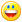Jaf Under
Registered User
Join date: 6 Jan 2005
Posts: 1
02-05-2005 22:51
amazing.
Adam Marker
new scripter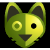Join date: 2 Jan 2004
Posts: 104
02-13-2005 14:58
So cool I'm speachl........
Adam Marker
new scripterJoin date: 2 Jan 2004
Posts: 104
02-13-2005 15:02
cool. so cool I'm speachl.......
Adam Marker
new scripterJoin date: 2 Jan 2004
Posts: 104
02-13-2005 15:05
cool. so cool I'm speachl....